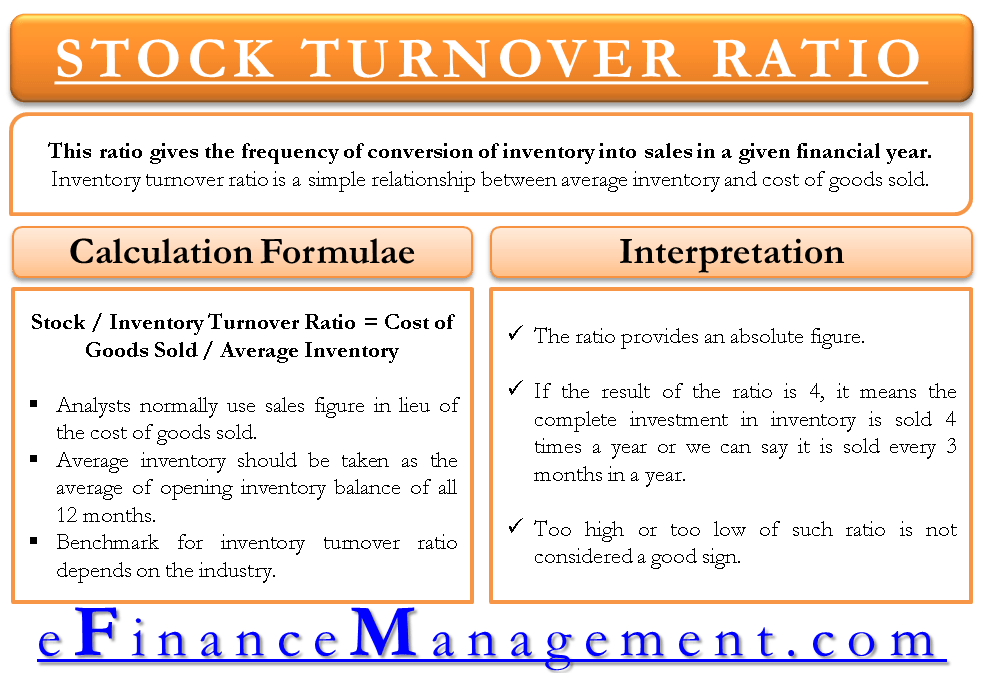# Inventory / Stock Turnover Ratio

Inventory turnover ratio is an important financial ratio to evaluate the efficiency and effectiveness of inventory management of the firm. This ratio indicates how many times inventory is sold and replaced in a financial year. In other words, the ratio gives the frequency of conversion of inventory into sales in a given financial year.

This ratio also talks of the effective utilization of the working capital, especially the capital that inventories block. Optimum utilization of working capital may reduce the working capital requirement and thereby save interest cost to the firm.

## How to Calculate Stock / Inventory Turnover Ratio?

Inventory turnover ratio is a simple relationship between average inventory and cost of goods sold. With these data in hand, the calculation of inventory turnover is very simple which is as follows:

## Formula for Inventory Turnover Ratio

Stock / Inventory Turnover Ratio = Cost of Goods Sold / Average InventoryThe dark side of the calculation is non-availability of required data i.e. Cost of Goods Sold and Average Inventory.

The cost of goods sold is normally not a part of financial statements which is a practical difficulty for an analyst. Analysts normally use sales figure in lieu of the cost of goods sold. Logically speaking, the ratio should not give correct result in that case and the reason is that the sales are based on market value and inventory is based on cost. So the two figures are not comparable to each other.

Average inventory should be taken as the average of opening inventory balance of all 12 months. This method will smoothen the seasonal effect in inventory levels and normalize the data. Like the cost of goods sold, availability of such detailed data for analysis is difficult many a times and therefore in the absence of that, an average of yearly opening and closing inventory is taken for the calculation.## Interpretation of the Ratio

Stock/inventory turnover ratio indicates how frequently the inventory is replaced. The ratio provides an absolute figure. Let’s understand with an example as to what it conveys. If the result of the ratio is 4, it means the complete investment in inventory is sold 4 times a year or we can say it is sold every 3 months in a year.

Normally, higher this ratio better is the inventory management. It is quite simple to understand. We have invested say ‘x’ amount of working capital in inventory. Interest cost on ‘x’ would remain fixed for the whole year irrespective of how many times that money is used to purchase inventory. So, it is obviously desirable to have higher ratios.  Higher frequency of turnover would mean effective utilization of working capital funds.

## What is Desirable – A Higher or a Lower Stock / Inventory Turnover Ratio?

Just like every other thing in life, a balance is also required here. Too high or too low of such ratio is not considered a good sign. Let’s see how.

Very High Inventory / Stock Turnover Ratio: Normally a higher ratio would be recommended as that would mean effective rolling up of working capital. At the same time, very high of such ratio would have a different meaning also. That may signal very low level of inventory being maintained. Too low inventory means too frequent orders. The manager should make sure that the additional ordering cost incurred should not outperform the savings of interest on working capital.

Very Low Inventory / Stock Turnover Ratio: Needless to explain, a very low turnover ratio of inventory will not utilize the fixed interest cost incurred on investment in inventory as explained in the above example.

## Benchmark or Ideal Ratio

Benchmark for inventory turnover ratio depends on the industry. A ratio which is considered good in one industry may be bad for the other. For example, FMCG goods would normally have higher inventory turnover ratio because the goods are cheap and are consumed very fast and on the top they are perishable also. On the other hand, big machinery which is costly in nature would always have a lower inventory turnover ratio. The best way to benchmark this ratio is to compare the concerned company ratio to the average of its respective industry in which it falls.

Last updated on : May 1st, 2019